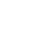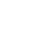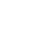# Glossary of Wave Terms

The Earth is a magnificent sphere that is full of constant motion. This constant motion is full to the brim with billions if not trillions of waves that work to carry energy and transport it from one location to another location.

People need to understand how waves transport energy, sound, color, light, smell, and everything else that the Earth provides. Understanding the physics of waves is best done by first getting familiar with its terminology. The physics of waves has a good number of terms such as:• Transverse Wave. Transverse Waves are Mechanical waves where their movement vibrates perpendicularly at a 90°(degree) angle to the direction that the energy is moving. Some examples of Transverse Waves are:

>Light Waves

>Infrared Waves

>X-Rays

>Ultraviolet Rays

• Longitudinal Waves. Longitudinal Waves are waves where the vibrations or oscillations move parallel to the direction that the wave of energy is travelling. A great example of Longitudinal waves is a soundwave.
• Waves are made up of peaks that consist of crests and troughs. The crest is the bump found on top of the wave. It is known as the upper most part of a wave that travels from one location to the next.
• As Crests are the bumps found on top of the wave,the Troughs are the bumps found at the bottom of the wave.
• When waves vibrate, they move towards a specific direction. They contain areas in their vibrations that do not move. These are called Nodes.
• Amplitude is the term that defines the maximum displacement from equilibrium, which is known as the highest point of a wave.
• Wavelength is the term used for defining the length and size of a wave
• Period is the term that defines the time it takes for a complete wave cycle to take place.
• Frequency is the term used in the measurement of the number of waves that passes by a certain point in a certain amount of time.

• The most common unit of frequency is known as a Hertz (Hz) and it is the measure of the number of waves that pass by a point in a second.
• Wavelength is characterized by the symbol (λ) and is the distance between consecutive corresponding points, which means one after the other. The measurement of the Wavelength of waves is in meters.
• Amplitude is characterized by the symbol (A) and considered the height of a wave where the measurement begins from the middle to its top.
• Frequency is characterized by the symbol (f) and is the number of full wavelengths produced in each second. The unit by which a Frequency is measured is known as a Hertz (Hz).
• Period is characterized by the symbol (T) and is the time measured for one complete wavelength to be produced.

Speed is characterized by the symbol (v) and it represents how quickly a wave transports energy from one location to the next.

• All sounds in the world can be represented by waves.
• Because of waves, people were able to create loudspeakers such as bullhorns.
• Because of waves, people were able to create noise-reduction headphones

### Can waves occupy the same space at the same time?

It is a fact that 2 solid objects cannot occupy the same space at the same time. Waves are a different matter as they are not matter. Because of this, waves are able to occupy the same space at the same time.

### How are waves formed in the ocean?

Waves are created when the wind blows across the sea, transferring its energy to the water.

### Why does the sky appear blue while space is black?

The sun’s rays produce white light that hits molecules in our atmosphere, which causes short blue wavelengths to scatter all over the sky.

•History
•Science
•Geography
•Biography
•US History